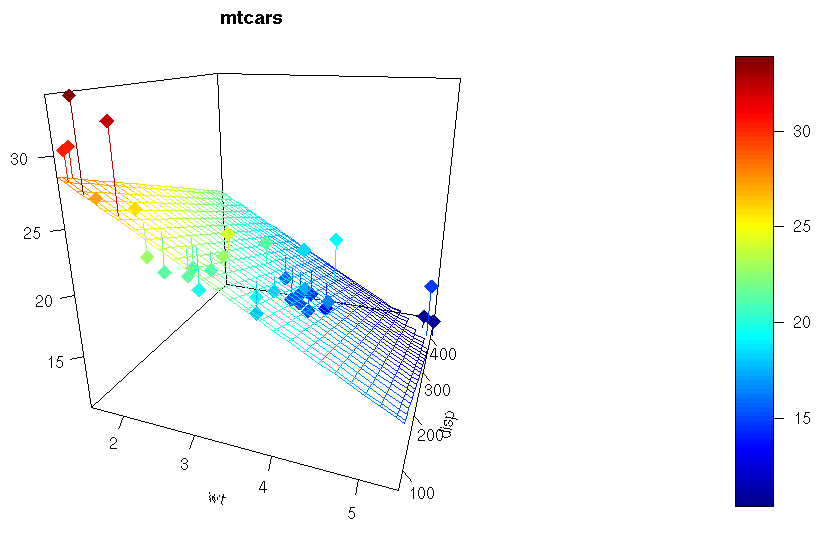• #5, First Floor, 4th Street Dr. Subbarayan Nagar Kodambakkam, Chennai-600 024 Landmark : Samiyar Madam
• pro@slogix.in
• +91- 81240 01111

### How to Visualize Regression Plane in R?

###### Description

To visualize the regression plane using R programming.

###### Process

R Function :lm()

To compute a linear regression model:ax + by + cz + d = 0

Argument surf To add a regression surface.

Surfis a list specifying a (fitted)surface to be added on the scatter plot.

The list should include at least x,y, z, defining the surface.

###### Sapmle Code

#Regression Plane

#Input

#x, y, z variables

x<-mtcars\$wt

y<-mtcars\$disp

z<-mtcars\$mpg

# Compute the linear regression (z = ax + by + d)

fit<-lm(z ~ x + y)

#Predict values on regular xy grid

grid.lines<-25

x.pred<-seq(min(x), max(x), length.out = grid.lines)

y.pred<-seq(min(y), max(y), length.out = grid.lines)

xy<-expand.grid( x = x.pred, y = y.pred)

z.pred<-matrix(predict(fit, newdata = xy), nrow = grid.lines, ncol = grid.lines)

#Fitted points for droplines to surface

fitpoints<-predict(fit)

#Scatter plot with regression plane

scatter3D(x, y, z, pch = 18, cex = 2,

theta = 20, phi = 20, ticktype = “detailed”,

xlab = “wt”, ylab = “disp”, zlab = “mpg”,

surf = list(x = x.pred, y = y.pred, z = z.pred,

facets=NA,fit = fitpoints), main = “mtcars”)

###### Screenshots